Area & Perimeter of Rhombus Calculator

Length of Rhombus
Enter Angle
Unit
Area  =  50in²
Perimeter  =  40in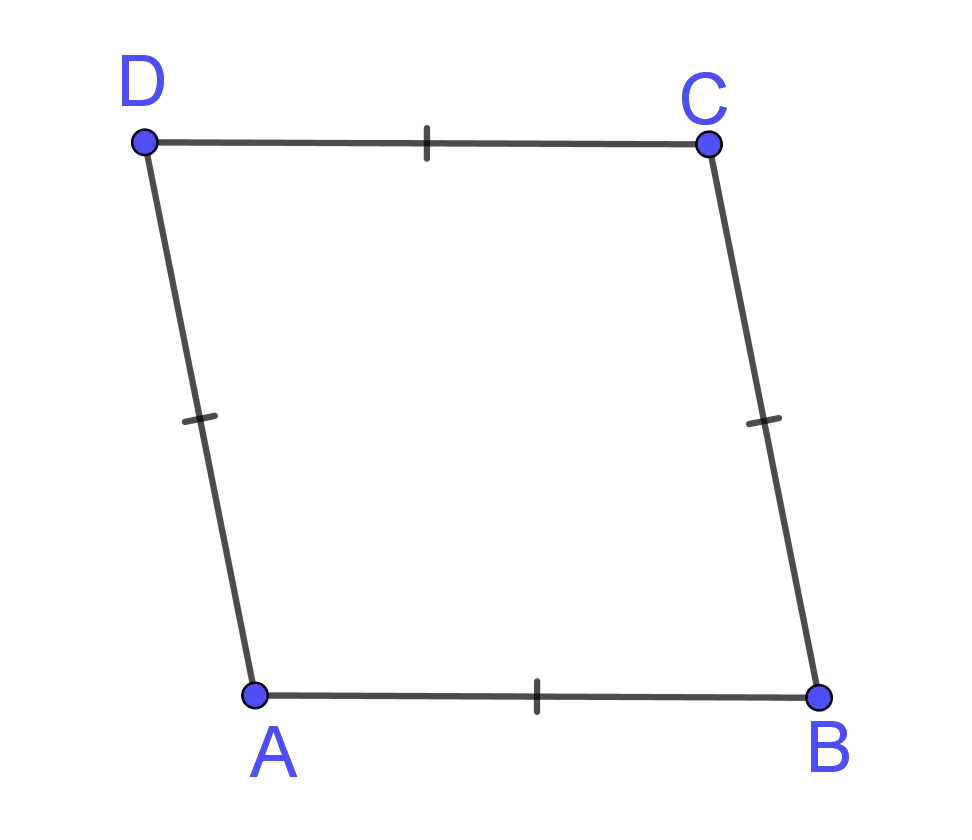GENERATE WORK

Area & Perimeter of Rhombus - work with steps

Input Data :
Length of Rhombus = 10 in
Angle = 30

Objective :
Find the perimeter of rhombus?

Formula
Area (Trigonometry) = A2 sinθ
Perimeter = 4 x Angle

Solution :
Area = 102 x sin(30)
= 100 x 0.5
Area = 50 in²

Perimeter = 4 x 30
Perimeter = 120 in

Area & Perimeter of Rhombus calculator uses side length and the measure of an angle or the lengths of diagonals of a rhombus, and calculates the perimeter and area of the rhombus. It is an online Geometry tool requires side length and measure of the angle or lengths of diagonals of a rhombus. Using this calculator, we will understand the trigonometric and diagonal method for finding the perimeter and area of a rhombus.
It is necessary to follow the next steps:

1. Enter the side length and the measure of an angle or the lengths of diagonals of a rhombus in the box. The length of a segment is always positive, so the values of the lengths of sides and diagonals must be positive real numbers. Since the angle of a rhombus is more than $0$ degrees and less than $180$ degrees, this value must be in the interval $(0,180)$ degrees.
2. Press the "GENERATE WORK" button to make the computation;
3. Rhombus calculator will give the perimeter and area of a rhombus.
Input (Trigonometric Method) :Two positive real numbers as the side length and the measure of an angle of a rhombus;
Input (Diagonal Method) :Two positive real numbers as the side length and the measure of an angle of a rhombus;
Output :Two positive real numbers as the perimeter and area of a rhombus and corresponding units after that.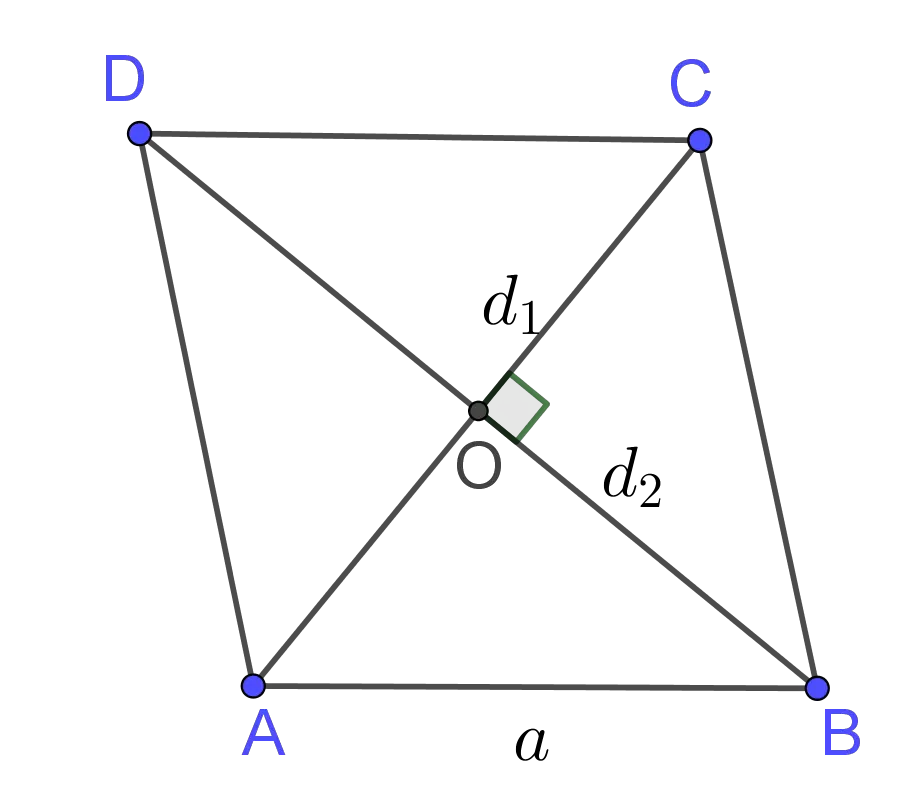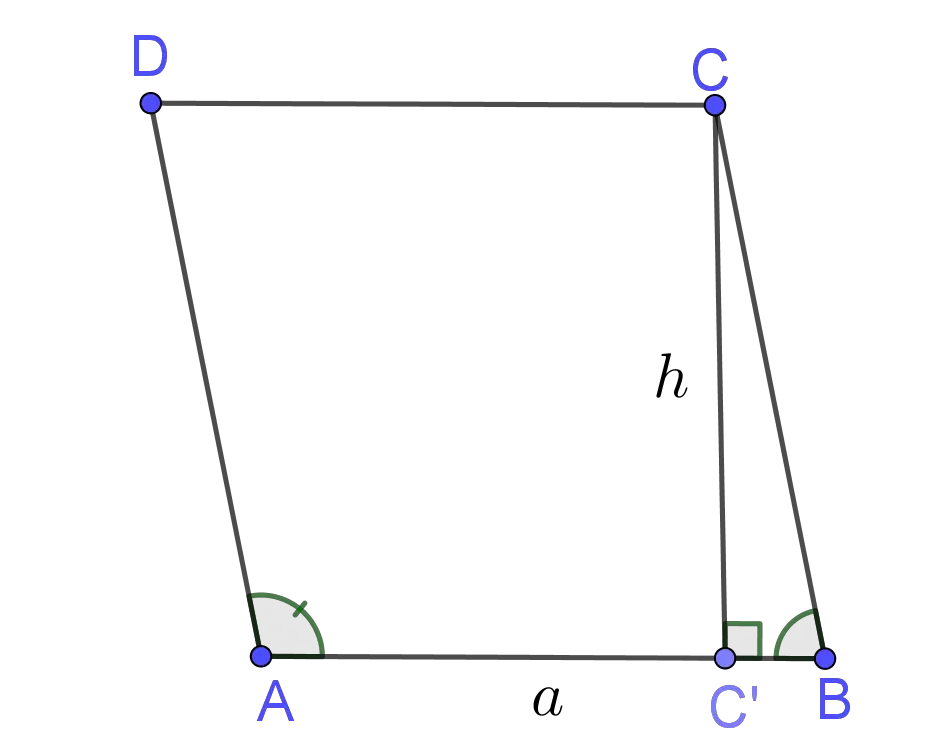Rhombus Formula :
Perimeter of Rhombus Formula:
The perimeter of a rhombus is determined by the following formula
$$P=4\times a$$ where $a$ is the length of the side of the rhombus.

Area of Rhombus Formula:
The area of a rhombus is determined by the following formula
$$A=a\times h$$ where $a$ and $h$ are lengths of the side and altitude of the rhombus, respectively.

Area of Rhombus Formula:
The area of a rhombus is determined by the following formula $$A=a^2\times \sin m\angle A=a^2\times \sin m\angle B$$ where $a$ is the length of the side of the rhombus.

Area of Rhombus Formula:
The area of a rhombus is determined by the following formula $$A=\frac 12d_1\times d_2$$ where $d_1$ and $d_2$ are lengths of the diagonals of the rhombus.

What is Area & Perimeter of Rhombus?

A quadrilateral with four congruent sides is a rhombus or diamond (see the picture below). In some literature it is named equilateral quadrilateral, since all of its sides are equal in length.That means, if ${\overline{AB}}\cong{\overline{BC}}\cong{\overline{CD}}\cong{\overline{DA}}$, then ${\overline{ABCD}}$ is a rhombus. Since opposite sides are parallel, the rhombus is a parallelogram, but not every parallelogram is a rhombus. That means that all the properties of a parallelogram can be also applied to rhombus. To recall, the parallelogram has the following properties:
• Opposite sides of a parallelogram are congruent;
• Opposite angles of a parallelogram are congruent;
• The consecutive angles of a parallelogram are supplementary to each other;
• The diagonals of a parallelogram bisect each other
Rhombus satisfies two more properties:
• The diagonals bisect the opposite angles of the rhombus;
• The diagonals of a rhombus are perpendicular.
Any quadrilateral with perpendicular diagonals, that one diagonal bisect other, is a kite. So, the rhombus is a kite, but not every kite is a rhombus. Any quadrilateral that is both a kite and parallelogram is a rhombus. Because the diagonals of a rhombus are perpendicular, we can apply the Pythagorean Theorem to find the rhombus side length. Let us consider the rhombus $\overline{ABCD}$. Diagonals of the rhombus bisect each other, $\overline{AO} \cong\overline{OC}$ and $\overline{BO}\cong\overline{OD}.$ Let us denote the side length and the lengths of diagonals of a rhombus by $a,d_1$ and $d_2$, respectively. Applying the Pythagorean theorem to $\Delta AOB$, we obtain
$${a}^2=\Big(\frac {d_1}2\Big)^2+\Big(\frac {d_2}2\Big)^2$$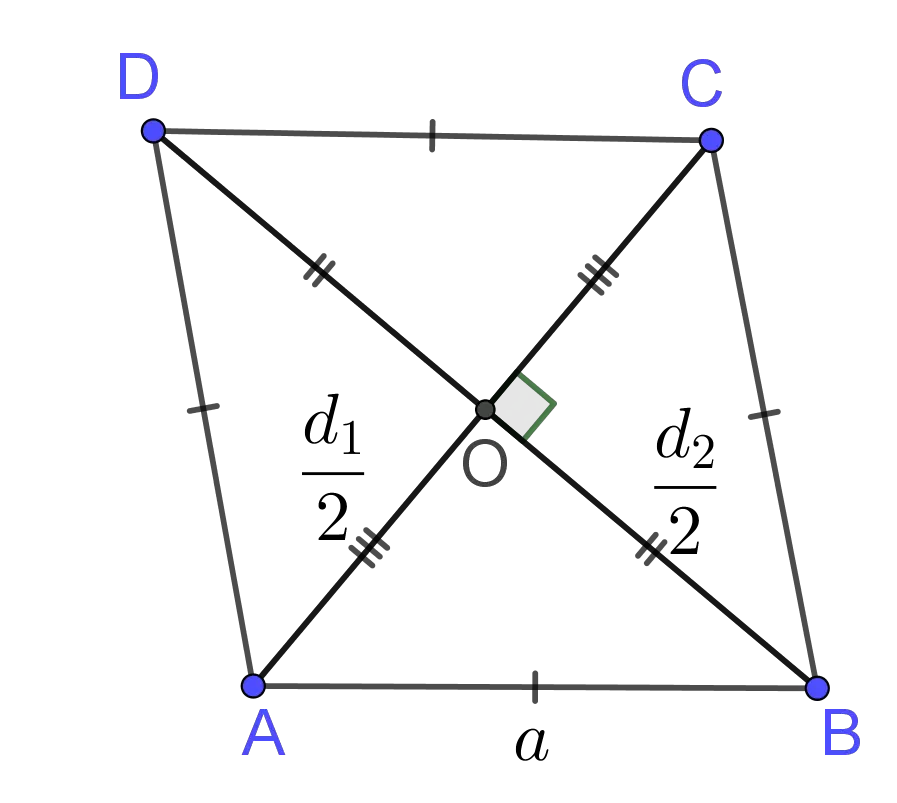The rhombus has only two lines of symmetry. A rhombus is symmetrical about its diagonals. A rhombus has central symmetry about the point of intersection of its diagonals, $O$. A rhombus has rotational symmetry because $180^o$ counterclockwise rotation about the point $O$ transforms the rhombus to itself.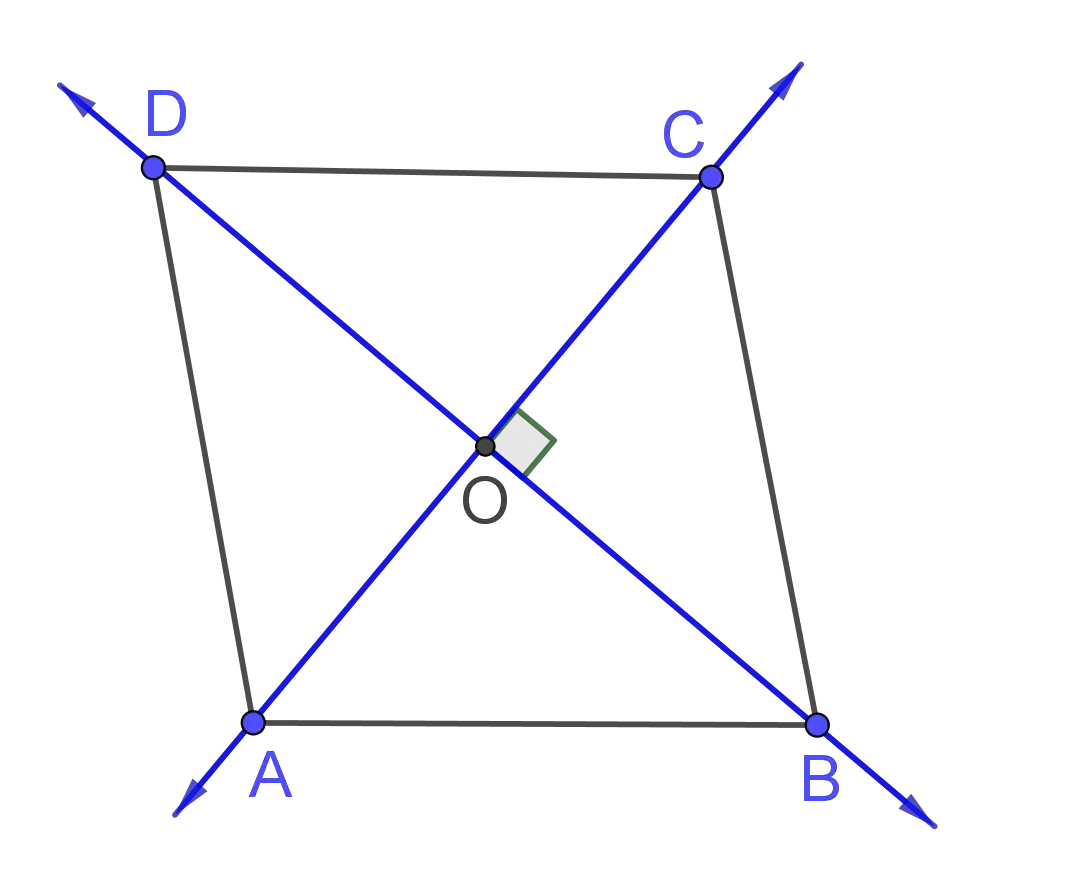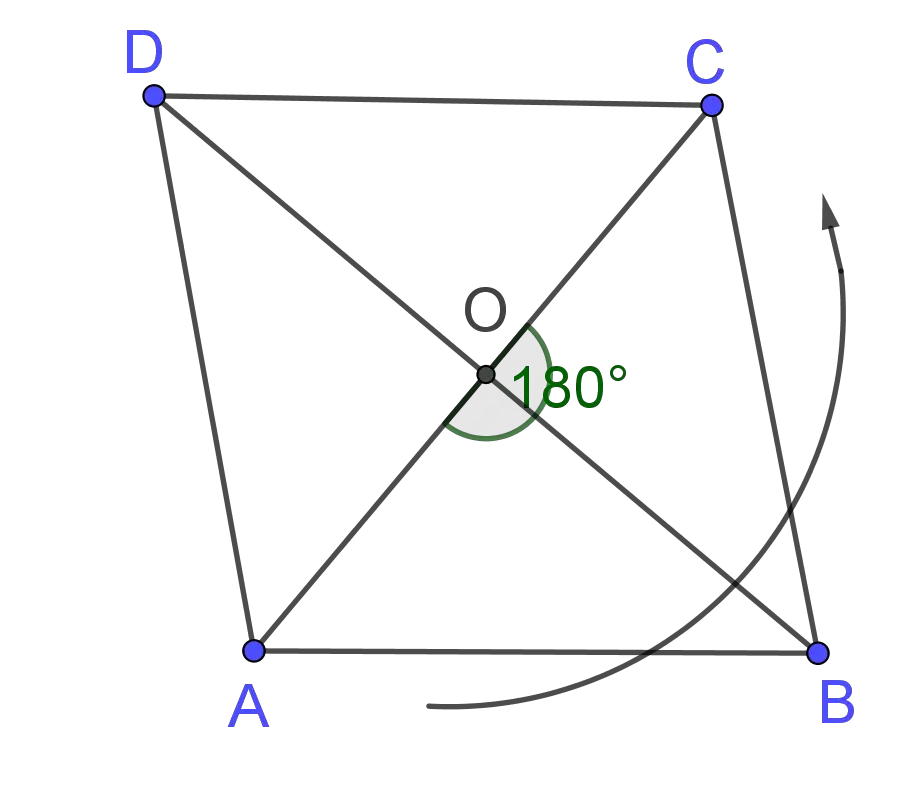The distance around a rhombus is called the perimeter of the rhombus. It is usually denoted by $P$. To find the perimeter of rhombus we add the lengths of its sides. Thus, the perimeter of a rhombus with side length of $a$ is
$$P =a+a+a+a= 4 \times a$$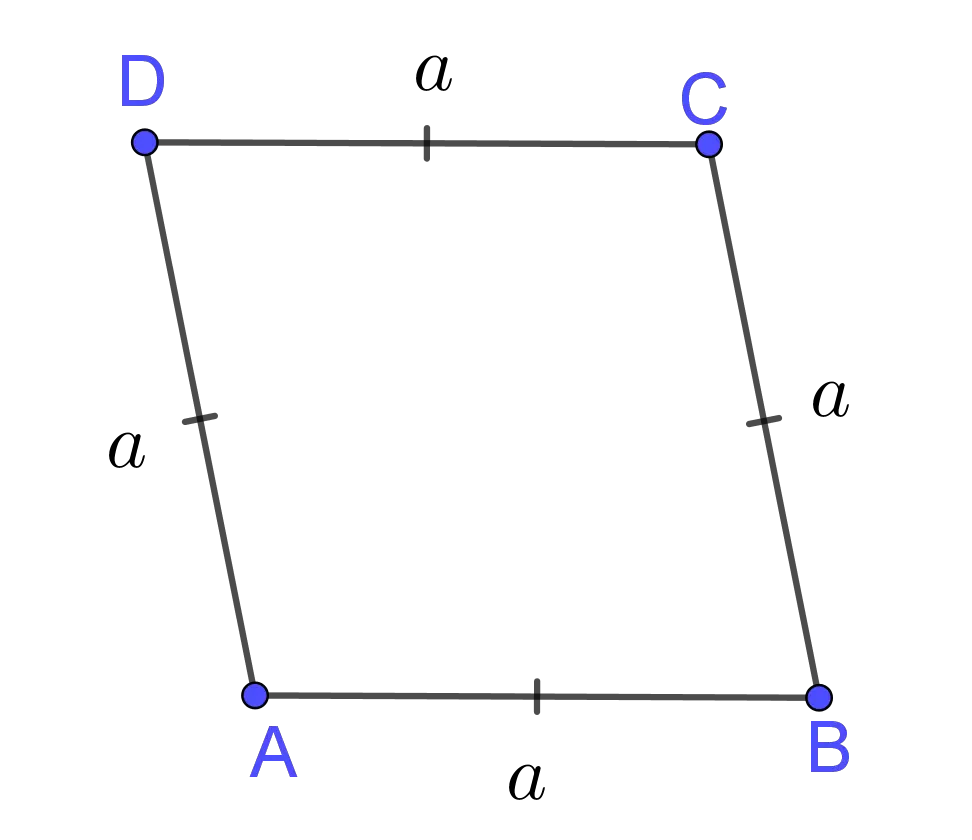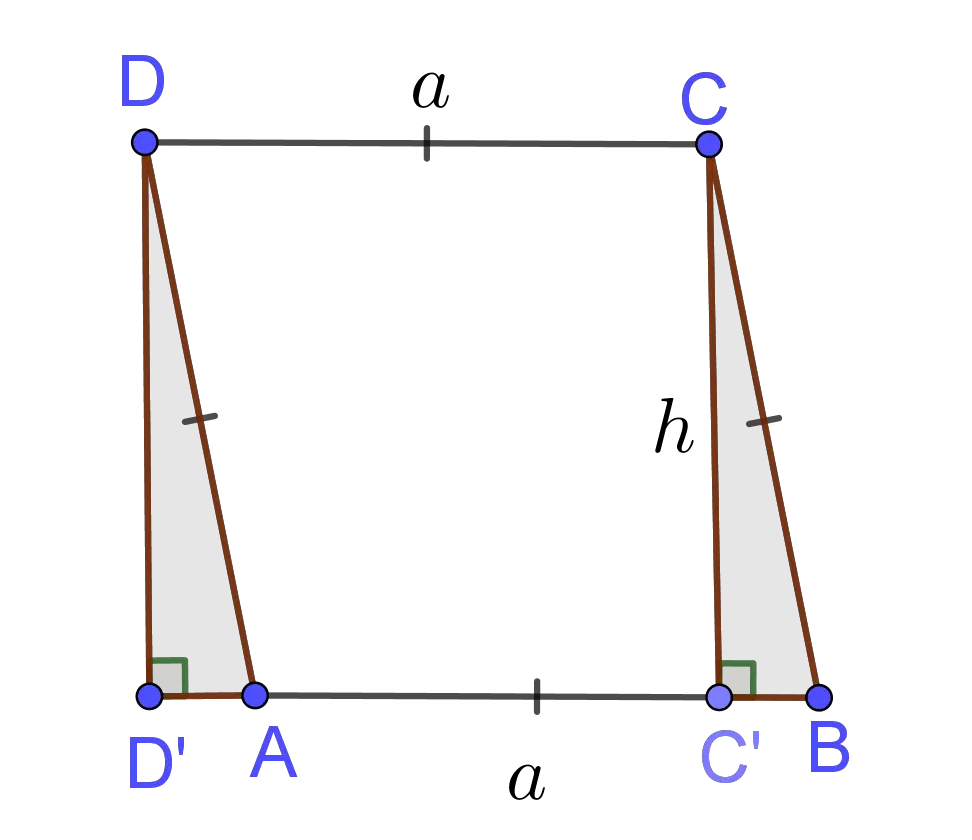The area of a rhombus is a number of square units needed to fill the rhombus. The area, usually denoted by $A$. A rhombus and a rectangle on the same base and between the same parallels are equal in area. Thus, area of the rhombus $\overline {ABCD}$ is equal to area of parallelogram $\overline{D'C'CD}$ (see picture on right). The area of a rhombus with the length of $a$ and the perpendicular distance between the opposite sides of $h$ is
$$A =a\times h$$
In other words, the area of a rhombus is the product of its base and height. If we use the sine ratio in the right triangle $\Delta CC'B$, $h=a\sin m\angle B$, the previous area formula becomes
$$A =a^2\times \sin m\angle B$$
Since $\sin(180^o-\alpha)=\sin \alpha$, $\angle A\cong\angle C$ and $\angle B\cong\angle D,$ we can use the measure of any angle in rhombus, i.e.
$$A =a^2\times \sin m\angle B=a^2\times \sin m\angle A$$
The area of a rhombus can be found in the second way. It is half the product of lengths of its diagonals.
$$A=\frac 12 d_1\times d_2$$
This formula follows from the formula for triangle area.The perimeter is measured in units such as centimeters, meters, kilometers, inches, feet, yards, and miles. The area is measured in units such as square centimeters $(cm^2)$, square meters $(m^2)$, square kilometers $(km^2)$ etc.

The area & perimeter of a Rhombus work with steps shows the complete step-by-step calculation for finding the perimeter and area of the rhombus with the side length of $10\;in$ and the measure of the angle of $30$ degrees using the perimeter and area formulas. For any other values for side length and measure of the angle of a rhombus, just supply two positive real numbers and click on the GENERATE WORK button. The grade school students may use this area and perimeter of a Rhombus to generate the work, verify the results of the perimeter and area of two-dimensional figures or do their homework problems efficiently.

Real World Problems Using Rhombus Metrics

Calculating areas and perimeters of various geometrical figures are very useful in mathematics as well as in real life problems. Rhombus often appear in real life. For instance, a square box, a cracker, a kite, a diamond, a pencil eraser are examples of rhombus.
In three-dimensional geometry, problems with prism and pyramid with rhombus bases very often appear. There is another interesting solid with rhombus bases, the so-called rhombic triacontahedron. It is the thirty-faced convex polyhedron with $30$ rhombic sides.

Rhombus Practice Problems

Practice Problem 1:
A rhombus has a perimeter of $60$ centimeters and the length of one diagonal is $16$ centimeters. Find its area.

Practice Problem 2:
If base angle of a rhombus measures $60$ degrees and the length of the side of the rhombus is $10\;in$, find its perimeter and area.

The Rhombus calculator, formula, example calculation (work with steps), real world problems and practice problems would be very useful for grade school students (K-12 education) to understand the concept of perimeter and area of rhombus. This concept can be of significance in geometry, to find the perimeter and area of complex figures, as well as to find surface area and volume of some solids.